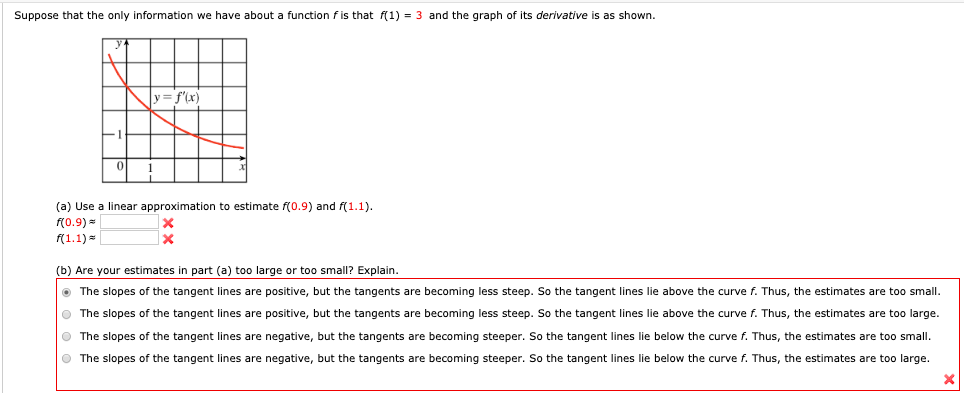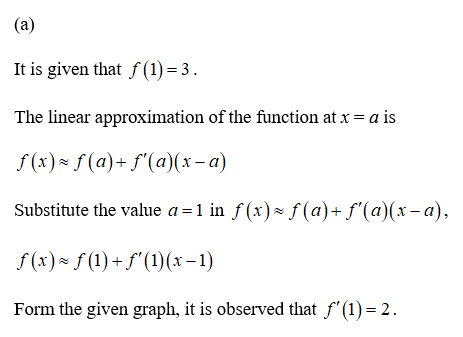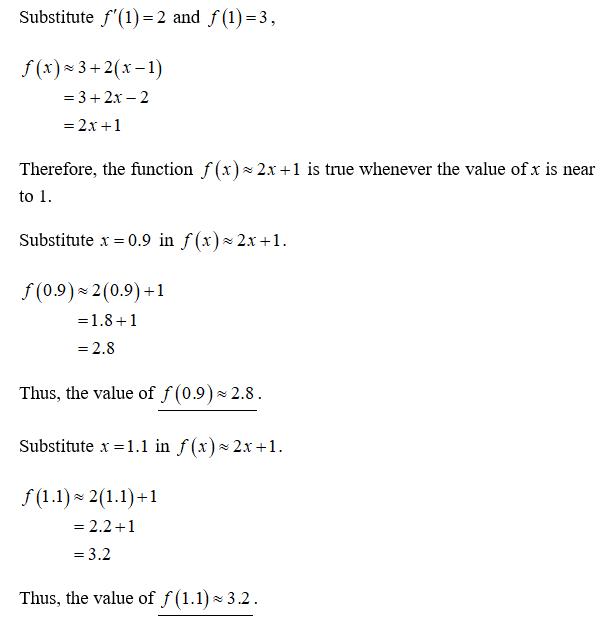# Suppose that the only information we have about a function fis that f(1) = 3 and the graph of its derivative is as shown.y=f'(x)(a) Use a linear approximation to estimate f(0.9) and f(1.1).f(0.9)f(1.1) =(b) Are your estimates in part (a) too large or too small? Explain.• The slopes of the tangent lines are positive, but the tangents are becoming less steep. So the tangent lines lie above the curve f. Thus, the estimates are too small.O The slopes of the tangent lines are positive, but the tangents are becoming less steep. So the tangent lines lie above the curve f. Thus, the estimates are too large.O The slopes of the tangent lines are negative, but the tangents are becoming steeper. So the tangent lines lie below the curve f. Thus, the estimates are too small.O The slopes of the tangent lines are negative, but the tangents are becoming steeper. So the tangent lines lie below the curve f. Thus, the estimates are too large.

Question
77 viewshelp_outlineImage TranscriptioncloseSuppose that the only information we have about a function fis that f(1) = 3 and the graph of its derivative is as shown. y=f'(x) (a) Use a linear approximation to estimate f(0.9) and f(1.1). f(0.9) f(1.1) = (b) Are your estimates in part (a) too large or too small? Explain. • The slopes of the tangent lines are positive, but the tangents are becoming less steep. So the tangent lines lie above the curve f. Thus, the estimates are too small. O The slopes of the tangent lines are positive, but the tangents are becoming less steep. So the tangent lines lie above the curve f. Thus, the estimates are too large. O The slopes of the tangent lines are negative, but the tangents are becoming steeper. So the tangent lines lie below the curve f. Thus, the estimates are too small. O The slopes of the tangent lines are negative, but the tangents are becoming steeper. So the tangent lines lie below the curve f. Thus, the estimates are too large. fullscreen
check_circle

Step 1Step 2...

### Want to see the full answer?

See Solution

#### Want to see this answer and more?

Solutions are written by subject experts who are available 24/7. Questions are typically answered within 1 hour.*

See Solution
*Response times may vary by subject and question.
Tagged in